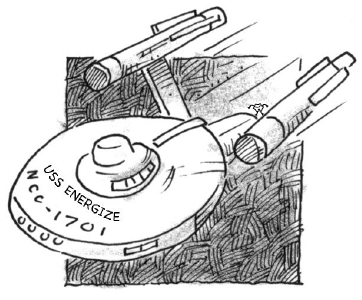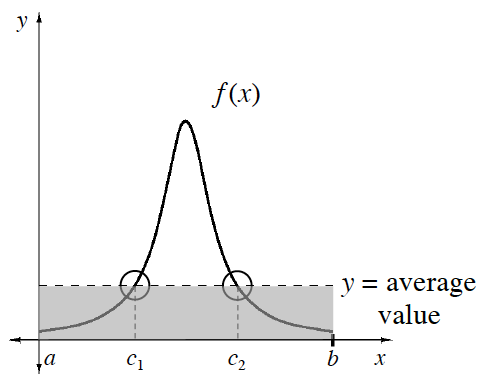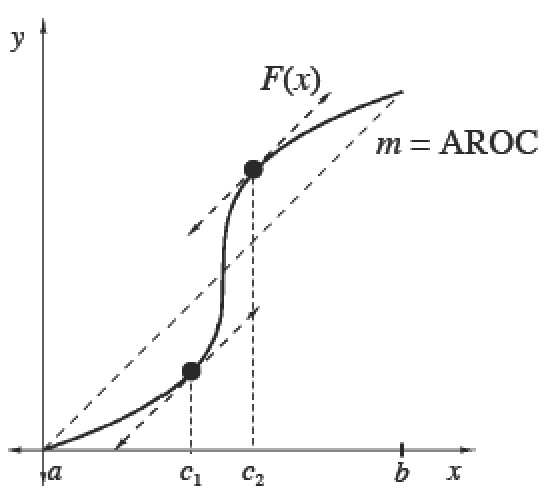### Home > APCALC > Chapter 8 > Lesson 8.4.1 > Problem8-145

8-145.

The function $v(t)$ below represents the velocity in kilometers per hour of the Starship Energize over a $20$-second period during which the warp-drive was engaged.

$v ( t ) = \left\{ \begin{array} { l l } { 10,000 + 100 t } & { \text { for } 0 \leq t < 10 } \\ { 10 ^ { t } } & { \text { for } 10 \leq t \leq 20 } \end{array} \right.$1. What was the average velocity of the starship during this interval?

$\text{average velocity}=\frac{\Delta \text{distance}}{\Delta \text{time}}$

You were given a velocity function. How will you find distance information?

2. At what time, if any, was the ship’s velocity equal to its average velocity? Does the Mean Value Theorem apply in this case? Explain.

The MVT states that if a function is both continuous and differentiable on a closed interval, then the average value will equal the actual value somewhere.

The Mean Value Theorem

 The Mean Value Theorem for IntegralsIf $f$  is continuous on $[a, b]$, then there exists at least one point $x = c$ in $\left(a, b\right)$ such that                     $\frac { 1 } { b - a } \int _ { a } ^ { b } f ( x ) d x= f(c)$.The Mean Value Theorem for DerivativesIf $F$ is continuous on $[a, b]$ and differentiable on $\left(a, b\right)$, then there exists at least one point $x = c$ in $\left(a, b\right)$ such that $F ^ { \prime } ( c ) = \frac { F ( b ) - F ( a ) } { b - a } = f ( c )$.3. What was the average acceleration of the starship during this interval?

$\text{Average Acceleration}=\frac{\Delta \text{velocity}}{\Delta \text{time}}$

Compare and contrast parts (a) and (c). Both are looking for averages. But, based on the function you were given, strategies to find averages are different.

4. At what time, if any, was the ship’s acceleration equal to this average acceleration? Does the Mean Value Theorem apply in this case? Explain.

MVT also states that if a function is both continuous and differentiable on a closed interval, then its average rate of change (AROC) will equal its actual rate of change (IROC) somewhere.